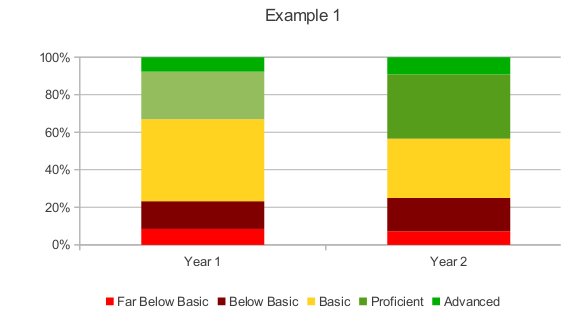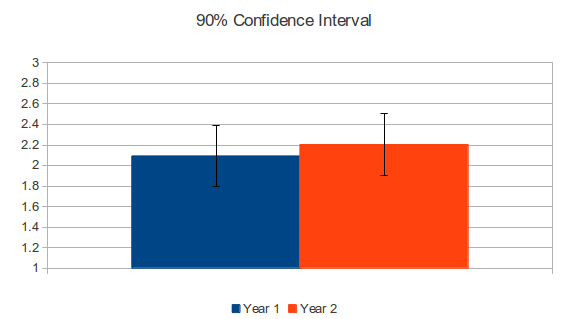# Can You Be Data Driven Without Statistics?

Have you ever seen a margin of error reported on a state test result or an error bar on a state test graph? Has anyone ever reported a p value, an R squared, a standard deviation, median, or any other statistical measure along with a test result? Frankly I can’t recall even seeing an average (mean) when state tests are discussed. If we are truly trying to be data driven in our decisions as educators and institutions, I believe we need to do some basic data analysis to understand this data or else we end up in a state of DRIP (Data Rich, Information Poor).

Scientists/statisticians will tell you that the result of a test is not a single, true number, but a number with an error margin (or confidence interval) around that number. So in political polls you will see 55%  +/- 3%, because we understand if we polled multiple times on the same day using the same polling method, we would get a variation in the end result. This is true for students taking tests as well, however the information about the amount of variance is unpublished. Why care? Because important decisions are based around whether or not test scores rise or fall. So a department in a school might be put under increased pressure if their scores fell by 5%. However, if the variance of the test is +/- 8% then a 5% change is insignificant. That is, it is not possible to say that the decrease in test scores is due to students actually knowing less or whether it is due to random chance and natural variation.

Error bars will increase with a smaller population size and with a wider range of results. So it is more difficult to make solid claims of change on a single class/department than an entire district and it is more difficult to make solid claims of change with a diverse group of abilities than on a group of students that have similar abilities.

Let’s look at an example of actual data, first without statistics, then with statistics. Here is a table and graph of test results:

 Year 1 Year 2 Change Far Below Basic 12 11 -1 Below Basic 21 27 6 Basic 62 48 -14 Proficient 36 52 16 Advanced 11 14 3

﻿This data suggests some improvement in test scores based on the “squint” analysis technique, i.e. getting a general impression based on the amount of green. In fact if you average the test scores on a 0=Far Below Basic to 4=Advanced scale you do see an improvement from 2.09 to 2.20 which is a 5% improvement.

However, to truly state the facts we have to include some information about the variance. Based on the standard deviation and population size, the 90% confidence interval for these averages is 14%, which means that the numbers actually have to be 14% greater in year 2 to show a significant increase. Another way of thinking about confidence intervals is that we are 90% certain the test results are +/- 14% from the reported value. When running a t-test to see if the two averages are significantly different,  the p value is .35, with .1 or less being considered significant in psychology. Here is a graph showing the average test scores with 90% confidence intervals for error bars.The large amount of overlap shows that there is no measurable difference between the two years, yet this sort of analysis is not regularly done. Based on the few tests I have looked at for my school’s variance and sample size, it seems that around a 15% difference is a significant difference. While there are certainly nuances of statistics I don’t understand, I could easily accomplish this analysis based on a single college stats course and a spreadsheet program (LibreOffice calc or Microsoft Excel). Instead of spending ten of thousands of dollars on data warehouse systems, schools should run their data through basic analysis and then only do further investigation on significant changes. Now that I know a 15% difference is the threshold for a real difference I can easily dismiss smaller variations as likely due to random noise. I would hope that administrators and board members would ask for this confidence interval for their schools and then use it as a filter before jumping to conclusions. I think it would surprise people at my school that even a 10% change from year to year is statistically insignificant. But that is the power of math, it reveals truths which are often counter intuitive. If we want to be data driven, we need to stop analyzing numbers with our gut and do the math.

PS- This example data does not show significant improvement in student learning, even given the assumption that a single 60 question multiple choice test is an accurate measure of student learning of a complex subject. If we start questioning the correlation between the results of the test and the actual student learning outcomes (success in college, career readiness, application of material learned in the real world), then we are even farther from being able to prove anything with these numbers.